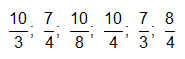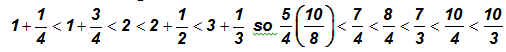# Fractions / Mixed Numbers

Activity 6 of 15
This lesson uses interactive number lines to help students investigate mixed numbers as a way to write improper fractions.

## Planning and Resources

Objectives
Students should understand that a proper fraction is a fraction whose numerator is less than the denominator and that an improper fraction is any fraction whose numerator is equal to or greater than the denominator.

Vocabulary
improper fraction
proper fraction
mixed number

Standard:

## Lesson Snapshot

#### Understanding

An improper fraction can be converted to the sum of a whole number and a fraction less than one. Converting a mixed number to a fraction can be thought of as fraction addition.

### What to look for

Lead students in a discussion about the relationship between the numerator and the denominator in an improper fraction.

### Sample Assessment

Order the following from smallest to largest.Answer: Thinking in mixed numbers is easier than fractions.### What are the students doing?

Division of a whole number by a smaller whole number results in an improper fraction that can be written as a mixed number.

### What is the teacher doing?

Have students work together to predict how the improper fraction will be expressed as a mixed number.